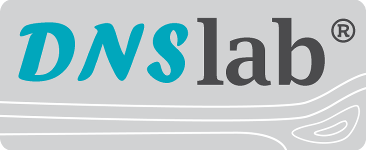Direct Numerical Simulation laboratory

## Filtration Efficiency

Assumed that the particle concentration is sufficiently small, the effect of the particles on the gas or liquid flow can be neglected as well as particle-particle interactions. Then the filtration efficiency of a porous medium can be computed with DNSlab by computing a steady-state flow field by numerical solution of the Navier-Stokes-equations and by subsequent computation of the particle trajectories and possible collisions with the porous structure. The ratio of the number of deposited particles to the total number of particles in a size fraction gives the fraction deposition rates.

## Publications

Publications on filtration efficiency computation with DNSlab can be found here >